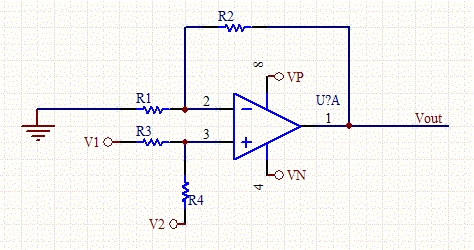## Non-Inverting Op-Amp Resistor Calculator

 Input Parameters (Non-Inverting Op-amp) Vout (V) Gain R1 (KOhms) V1 (Input lead) (V) V2 (non-zero only if offset is requred) (V) Vp (V) Vn (V) Outputs R2 (KOhms) R3 (KOhms) R4 (KOhms)Give the gain and desired output bias point to determine the bias and feedback resistors for a non-inverting op-amp.

Thinkcalculator.com provides you helpful and handy calculator resources.